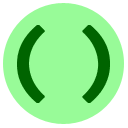# 12: Tips

This lesson contains a few facts about the Python language which we left out of earlier lessons.

## `print` Options

So far, the only way we have seen to print is to use the `print()` function, which prints several items one line at a time, and separates all items by spaces. In fact, print accepts a few keyword arguments which allow you to modify this behaviour to suit your needs.

• To change the space separator to some other string `S`, include the argument `sep = «S»`
• To replace the "end-of-line" character with some other string `S`, include the argument `end = «S»`

This new feature, keyword arguments, is easiest to demonstrate with a few examples.

Example
Examples of `sep` and `end`. Look at the effect of each `print` call.

These keyword arguments must be passed at the end of the argument list or else an error will occur. If you use both they can be given in any order.Coding Exercise: Alphabet Aerobics
Using what we just introduced, debug the following program so that it outputs the upper-case alphabet all on one line.

## Valid Variable/Function Names

• Names can contain letters, numbers, and the underscore (`_`) character.
• The first character of every name must be a letter.
• Python distinguishes between upper- and lower-case letters.

Thus `my_3rd_int` is a valid variable name, but `3rd_int` is not.

Example
An example of distinguishing between upper- and lower-case.

## Descending `for` loops & other increments

Recall that we showed how `for` loops can iterate through numbers in increasing order:

Example
A `for` loop

Quite often, it is necessary to write a `for` loop which goes through the numbers in descending order (biggest to smallest). To do this we call `range` with a third argument called the step:

Example
Negative step for `range`

If you think for a moment, you also notice `range(0, 5)` is the same as `range(0, 5, 1)`: the default increment is 1. In either case, be careful that `range(start, stop, step)` goes until just before reaching the value `stop`. For more information you can see the Python manual.Coding Exercise: Lucky Sevens
Write a program, using a `for` loop with step size 10, to print out all 2-digit numbers which end in 7, in increasing order.

Similarly, you can use `string[x:y:2]` to get the substring with characters `x, x+2, x+4, ...` or `string[y:x:-1]` to get a reversed part of a string (where `y` > `x`).

With a `for` loop, another way to accomplish a decreasing range is `reversed(range(x, y))`, which goes from `y-1` to `x` in decreasing order.

## Writing Smaller Code

Python allows several ways to write shorter code; we introduce a few of them here.On this website we won't always use these features, since sometimes it makes code harder to read.

### Assignment Operators

Python lets you write "`x += 1`" to mean "add one to `x`." So it is equivalent to "`x = x + 1`" as we show below:

Example
Examples of `+=`, `-=`, `*=`, `/=`

Similarly, there are operators for integer division (`//=`), modulus (`%=`), and power (`**=`).

### Inline Blocks

We have seen several statements which are followed by indented "blocks" of code: `for`, `if`, `else`, `elif`, `while`, and `def`, for example:

```if x==y:
«block» #indented, multiline```
In the special case that the `«block»` is only one line long, Python allows the following alternative syntax:

`if x==y: «block» #single line`
Here is an example:

Example
Inline block statements

This has its limits however: a compound block with a colon (`if`, `for`, etc) can't be used as an inline block. For example, `if 2>1: if 4>3: print()` gives a syntax error.

### Multiple Assignments

Python allows you to combine two assignment statements into one:

Example
Multiple assignment

Note that this allows you to solve our swapping exercise using only one line!

### Comparison Chaining

Python also allows you to combine several comparisons into one:

Example
Multiple assignment
Any chain `v1 c1 v2 c2 v3 ...` where `v` are values and `c` are comparisons, is treated the same as `(v1 c1 v2) and (v2 c2 v3) and...`

### Default values for `[:]` and `range()`

You can leave out one or both of the start/end values when using the sub-string operator `[:]`. (This is also true for lists, as we'll see in the next lesson.) The default value of the first index is `0` (the start of the string) and the default value of the last index is the length of the string. Likewise, `range(n)` is short for `range(0, n)`.

Example
Using the default values for `[:]` and `range()`

# `None`

Some functions return a value as their main effect, like `abs(x)`. But other functions which are more valuable for their effects, like `print()`, still return the 'default' value `None`:

Example
The value of `print`

Here `None` is a special value used by Python as a general-purpose placeholder. If you call type(None), you find out that `None` even has its own type, `NoneType`. While we are at it, let's see what is the type of a type variable:

Example
The type of a type

In the next exercise, we ask you to carefully track the results of a long command using `type` and `print`.Scramble Exercise: One None
The output of `print(type(print(type(type(print(print()))))))` consists of 4 lines. Put them in the correct order.
• <class 'NoneType'>
• «blank line»
• <class 'type'>
• None
Correct!

That is all of the tips for now. You are ready to continue to the next lesson!